### Home > A2C > Chapter Ch11 > Lesson 11.3.3 > Problem11-157

11-157.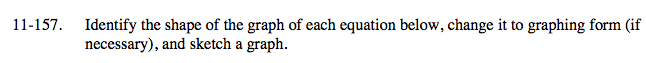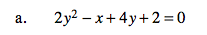2y2 + 4y + 2 = x

x = 2(y + 1)2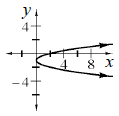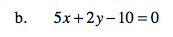2y = −5x + 10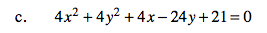Try to factor and complete the squares for x and y.

4(x2 + x) + 4(y2 − 6y) = −21

$4\left(x^2+x+\frac{1}{4}\right)+4(y^{2}-6y+9)$

= − 21 + 1 + 36

$\left(x+\frac{1}{2}\right)^2+(y-3)^2=4$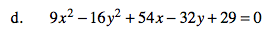See part (c).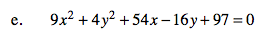See part (c).

9(x2 + 6x) + 4(y2 − 4y) + 97 = 0

9(x2 + 6x + 9) − 81 + 4(y2 − 4y + 4) − 16 + 97 = 0

9(x + 3)2 + 4(y − 2)2 = 0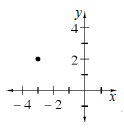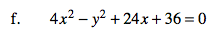See part (c).

Notice that y 2 is the only term containing y. Try to get it by itself.